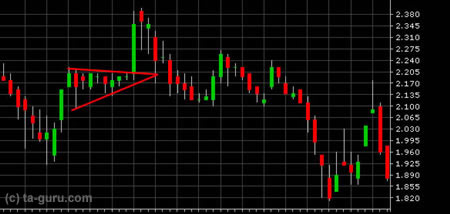Chart Pro online
User name (email):

 Recently used

 Forex

 Indices

# Triangles

Triangles belong to continuation patterns. They are used as an early indicator for greater price moves. Edges of the triangle are formed by two lines representing the support and resistance. On one side they are intersected and on the other side they could be connected by base, so triangle could be drawn.

Within the triangle, prices are changing, and they are touching triangle edges several times. As the triangle is formed, variations are lower as well as trading volume. An interesting phenomenon that occurs is a rule of five: price will move from one edge to another five times before it penetrates one of them. If the price penetrates triangle edge by 3%, penetration is confirmed. After that significant price change is expected.

Breakthrough of triangle edge must be confirmed by volume, so volume must be increased during penetration. Price change is expected to be proportional to the size of the triangle base.

There are three types of triangles: symmetrical, upward and downward.Symmetrical triangle

Symmetrical triangle consists of two intersected converging trend lines. Since drawing of trend line requires two points, drawing of symmetrical triangle requires a minimum of four points.

When triangle is formed, there is a time limit for breakthrough. Because as time passes the amplitude of price oscillation is reduced due to movement towards the apex. Penetration usually occurs somewhere between two thirds and three fourths of the width of the triangle. Width of the triangle is measured from the base to the apex.

After a penetration, it could happen that price return to level of broken trend line. In case of upward trend broken trend line can become line of support, or line of resistance in case of downward trend.

Volume during penetration should be increased, otherwise pattern could not be confirmed. If price returns to level of broken trend line, it must seen off with lower volume. In an upward trend it is desirable that price increases in the triangle with greater volume and to decrease with lower volume. Opposite is true for downward trend.Parallel line method

The level to which prices will move can be calculated using the base of the triangle. In an upward trend, projection of base line to the point of penetration can be used for measuring price movement. Another method is method of parallel line: draw parallel line to triangle edge from point of base line apex.

Ascending triangle occurs primarily in the upward trend. It differs from symmetric triangle because it has upper edge almost parallel to the x axis. It is consequence of presence of aggressive buyers.

Ascending triangle behaves identical to the symmetrical triangle. Time for penetration is limited and penetration must be confirmed by volume. The level to which prices will move is calculated in the same way.

Descending triangle is opposite of ascending triangle: it has lower edge almost parallel to the x axis, because of aggressive sellers. It occurs in the downward trend.

Although the ascending triangle usually occurs in the upward trend, and descending in the downward trend, opposite situation can also happen. In that case, the triangle indicates a trend reversal.Ascending triangle